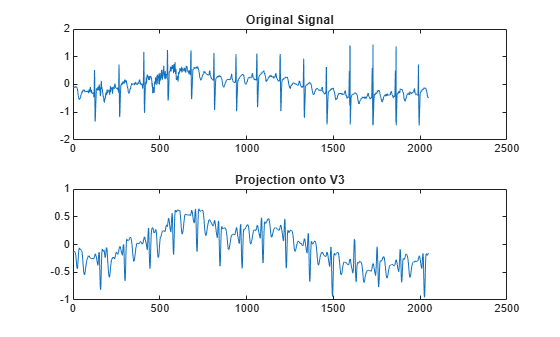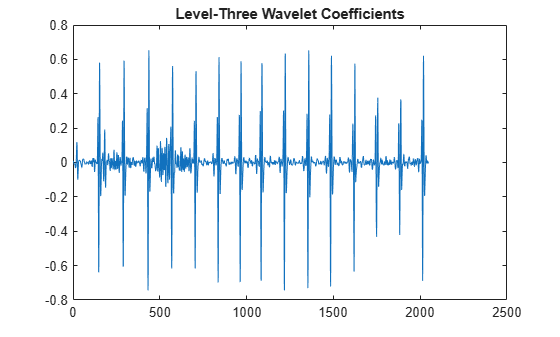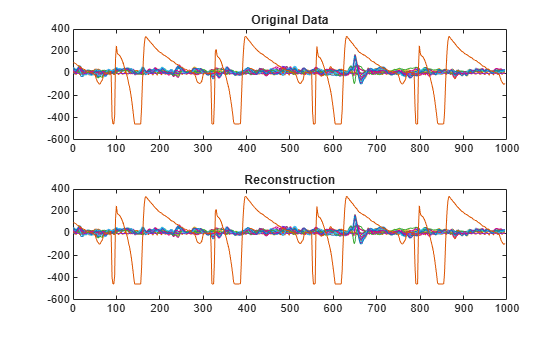# imodwt

Inverse maximal overlap discrete wavelet transform

## Syntax

``xrec = imodwt(w)``
``xrec = imodwt(w,wname)``
``xrec = imodwt(w,Lo,Hi)``
``xrec = imodwt(___,lev)``
``xrec = imodwt(___,'reflection')``

## Description

example

````xrec = imodwt(w)` reconstructs the signal based on the maximal overlap discrete wavelet transform (MODWT) coefficients in `w`. By default, `imodwt` assumes that you obtained `w` using the `'sym4'` wavelet with periodic boundary handling. If you do not modify the coefficients, `xrec` is a perfect reconstruction of the signal.```

example

````xrec = imodwt(w,wname)` reconstructs the signal using the orthogonal wavelet `wname`. `wname` must be the same wavelet used to analyze the signal input to `modwt`.```

example

````xrec = imodwt(w,Lo,Hi)` reconstructs the signal using the orthogonal scaling filter `Lo` and the wavelet filter `Hi`. The `Lo` and `Hi` filters must be the same filters used to analyze the signal input to `modwt`.```

example

````xrec = imodwt(___,lev)` reconstructs the signal up to level `lev`. `xrec` is a projection onto the scaling space at level `lev`. The default level is 0, which results in perfect reconstruction if you do not modify the coefficients.```

example

````xrec = imodwt(___,'reflection')` uses the reflection boundary condition in the reconstruction. If you specify `'reflection'`, `imodwt` assumes that the length of the original signal length is one half the number of columns in the input coefficient matrix. By default, ` imodwt` assumes periodic signal extension at the boundary.You must enter the entire character vector `'reflection'`. If you added a wavelet named `'reflection'` using the wavelet manager, you must rename that wavelet prior to using this option. `'reflection'` may be placed in any position in the input argument list after `x`.```

## Examples

collapse all

Obtain the MODWT of an ECG signal and demonstrate perfect reconstruction.

Load the ECG signal data and obtain the MODWT.

`load wecg;`

Obtain the MODWT and the Inverse MODWT.

```w = modwt(wecg); xrec = imodwt(w);```

Use the L-infinity norm to show that the difference between the original signal and the reconstruction is extremely small. The largest absolute difference between the original signal and the reconstruction is on the order of $1{0}^{-12}$, which demonstrates perfect reconstruction.

`norm(abs(xrec'-wecg),Inf)`
```ans = 2.3255e-12 ```

Obtain the MODWT of Deutsche Mark-U.S. Dollar exchange rate data and demonstrate perfect reconstruction.

Load the Deutsche Mark-U.S. Dollar exchange rate data.

`load DM_USD;`

Obtain the MODWT and the Inverse MODWT using the `'db2'` wavelet.

```wdm = modwt(DM_USD,'db2'); xrec = imodwt(wdm,'db2');```

Use the L-infinity norm to show that the difference between the original signal and the reconstruction is extremely small. The largest absolute difference between the original signal and the reconstruction is on the order of $1{0}^{-13}$, which demonstrates perfect reconstruction.

`norm(abs(xrec'-DM_USD),Inf)`
```ans = 1.6370e-13 ```

Obtain the MODWT of an ECG signal using the Fejer-Korovkin filters.

`load wecg;`

Create the 8-coefficient Fejer-Korovkin filters.

`[Lo,Hi] = wfilters('fk8');`

Obtain the MODWT and Inverse MODWT.

```wtecg = modwt(wecg,Lo,Hi); xrec = imodwt(wtecg,Lo,Hi);```

Plot the original data and the reconstruction.

```subplot(2,1,1) plot(wecg) title('ECG Signal'); subplot(2,1,2) plot(xrec) title('Reconstruction')```Obtain the MODWT of an ECG signal down to the maximum level and obtain the projection of the ECG signal onto the scaling space at level 3.

`load wecg;`

Obtain the MODWT.

`wtecg = modwt(wecg);`

Obtain the projection of the ECG signal onto ${V}_{3}$, the scaling space at level three by using the `imodwt` function.

`v3proj = imodwt(wtecg,3);`

Plot the original signal and the projection.

```subplot(2,1,1) plot(wecg) title('Original Signal') subplot(2,1,2) plot(v3proj) title('Projection onto V3')```Note that the spikes characteristic of the R waves in the ECG are missing in the ${V}_{3}$ approximation. You can see the missing details by examining the wavelet coefficients at level three.

Plot the level-three wavelet coefficients.

```figure plot(wtecg(3,:)) title('Level-Three Wavelet Coefficients')```Obtain the inverse MODWT using reflection boundary handling for Southern Oscillation Index data. The sampling period is one day. `imodwt` with the `'reflection'` option assumes that the input matrix, which is the `modwt` output, is twice the length of the original signal length. `imodwt` reflection boundary handling reduces the number of wavelet and scaling coefficients at each scale by half.

```load soi; wsoi = modwt(soi,4,'reflection'); xrecsoi = imodwt(wsoi,'reflection');```

Use the L-infinity norm to show that the difference between the original signal and the reconstruction is extremely small. The largest absolute difference between the original signal and the reconstruction is on the order of $1{0}^{-11}$, which demonstrates perfect reconstruction.

`norm(abs(xrecsoi'-soi),Inf)`
```ans = 1.6421e-11 ```

Load the 23 channel EEG data `Espiga3` . The channels are arranged column-wise. The data is sampled at 200 Hz.

`load Espiga3`

Obtain the maximal overlap discrete wavelet transform down to the maximum level.

`w = modwt(Espiga3);`

Reconstruct the multichannel signal. Plot the original data and reconstruction.

```xrec = imodwt(w); subplot(2,1,1) plot(Espiga3) title('Original Data') subplot(2,1,2) plot(xrec) title('Reconstruction')```## Input Arguments

collapse all

MODWT transform of a signal or multisignal down to level L, specified as a matrix or 3-D array, respectively. `w` is an L+1-by-N matrix for the MODWT of an N-point signal, and an L+1-by-N-by-NC array for the MODWT of an N-by-NC multisignal. By default, `imodwt` assumes that you obtained the MODWT using the `'sym4'` wavelet with periodic boundary handling.

Data Types: `single` | `double`
Complex Number Support: Yes

Synthesis wavelet, specified as one of the following:

• `'haar'` — Haar wavelet

• `'dbN'` — Extremal phase Daubechies wavelet with `N` vanishing moments, where `N` is a positive integer from 1 to 45.

• `'symN'` — Symlets wavelet with `N` vanishing moments, where `N` is a positive integer from 2 to 45.

• `'coifN'` — Coiflets wavelet with `N` vanishing moments, where `N` is a positive integer from 1 to 5.

• `'fkN'` — Fejér-Korovkin wavelet with `N` coefficients, where `N = 4, 6, 8, 14, 18` and `22`.

The synthesis wavelet must be the same wavelet used in the analysis with `modwt`.

Filters, specified as a pair of even-length real-valued vectors. `Lo` is the scaling filter, and `Hi` is the wavelet filter. `Lo` and `Hi` must be the same filters used in the analysis with `modwt`. The filters must satisfy the conditions for an orthogonal wavelet. The lengths of `Lo` and `Hi` must be equal. See `wfilters` for additional information. You cannot specify both a wavelet `wname` and filter pair `Lo,Hi`.

Reconstruction level, specified as a nonnegative integer between 0 and `size(w,1)-2`. The level must be less than the level used to obtain `w` from `modwt`. If `lev` is 0 and you do not modify the coefficients, `imodwt` produces a perfect reconstruction of the signal.

## Output Arguments

collapse all

Reconstructed version of the original signal or multisignal based on the MODWT and the level of reconstruction, returned as a vector or matrix.

 Percival, Donald B., and Andrew T. Walden. Wavelet Methods for Time Series Analysis. Cambridge Series in Statistical and Probabilistic Mathematics. Cambridge ; New York: Cambridge University Press, 2000.

 Mesa, Hector. “Adapted Wavelets for Pattern Detection.” In Progress in Pattern Recognition, Image Analysis and Applications, edited by Alberto Sanfeliu and Manuel Lazo Cortés, 3773:933–44. Berlin, Heidelberg: Springer Berlin Heidelberg, 2005. https://doi.org/10.1007/11578079_96.

## SupportGet trial now